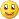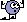# Requests & Ideas - page 249355

Wintersky I am failing to find that part of the code in FGDI. Can you pint me out more precisely where should I find it?

It's at Line 247 in MetaEditor of the FGDI code. Need you to insert the median right in between Line 247 and Line 248, as shown below

----------------------------------------------------------------------

delta=MathSqrt( MathPow( diff - priorDiff, 2.0)+(1.0/MathPow( e_period, 2.0)) ); sum+=MathPow(delta-(length/g_period_minus_1),2);

----------------------------------------------------------------------

Yours sincerely,

Wintersky163284

wintersky111:

It's at Line 247 in MetaEditor of the FGDI code. Need you to insert the median right in between Line 247 and Line 248, as shown below

----------------------------------------------------------------------

delta=MathSqrt( MathPow( diff - priorDiff, 2.0)+(1.0/MathPow( e_period, 2.0)) ); sum+=MathPow(delta-(length/g_period_minus_1),2);

----------------------------------------------------------------------

Yours sincerely,

Wintersky

Wintersky

Looking at that part of the code and this is what is my conclusion so far

fdi=1.0 +(MathLog( length)+ LOG_2 )/MathLog( 2 * g_period_minus_1 );

mean=length/g_period_minus_1;

for( iteration=0; iteration <= g_period_minus_1; iteration++ )

{

if(( priceMax - priceMin)> 0.0 )

{

diff =(inputData[pos + iteration] - priceMin )/( priceMax - priceMin );

if(iteration > 0 )

{

delta=MathSqrt( MathPow( diff - priorDiff, 2.0)+(1.0/MathPow( e_period, 2.0)));

//

//

// median would be limited to g_period_minus_1 length

// but in increasing lengths : 1 to g_period_minus_1

// due to how an array of intermediate results would be filled in

//

//

//

sum+=MathPow(delta-(length/g_period_minus_1),2);

}

priorDiff=diff;

}

}

variance=sum/(MathPow(length,2)*MathPow(MathLog(2*g_period_minus_1),2));

It is simply a promblem that the sample for fdi calculation is a variable length sample. I can make it calculate as a median of that variable length (I am assuming that it would be a median of delta values) or we should already use a median in the moment when inputData[pos + iteration] is used as an input for calculation. Let me know what dio you think355

It is simply a promblem that the sample for fdi calculation is a variable length sample. I can make it calculate as a median of that variable length (I am assuming that it would be a median of delta values) or we should already use a median in the moment when inputData[pos + iteration] is used as an input for calculation. Let me know what dio you think

I was thinking about this issue too even before you raised it and was quite in a bind about what to think about it. The issue wouldnt be there if N or periods tended towards infinity certainly.

Either way of calculation, the difference (if any) shouldn't be a really major issue. That and the fact that FD value itself is still more of a general value rather than a precise value, given that it is but an estimation (due to noise, nonlinearities etc etc). & that is the viewpoint expressed by most papers of the difficulty of giving a good precise FD value.

So either way is fine mladen. Or if you feel that one way of placing the median is better than the other, i am fine with whichever you choose. Thanks.

Yours sincerely,

Wintersky60

Good evening, Mladen ! I would like to make a new request about the option of upgrading

the indicator from mq4 into mq5 ( it’s another month already !)

As always it’s from the arsenal that developed by you.

Files:163284

MikhaelPankov:
Good evening, Mladen ! I would like to make a new request about the option of upgrading

the indicator from mq4 into mq5 ( it’s another month already !)

As always it’s from the arsenal that developed by you.

Mikhael

Here you goMulti time frame + alerts and the fill area that is used is specific to metatrader 5 (at least that is nicer than in the metatrader 4 version)

Files:60

Dear Mladen ! A mountain of thanks - it's simply exciting !

Full of respect, Mikhael.383

Idea

Good afternoon everyone:

mladen, first I apologize for creating threads without good reason, as they say in my country, the rush is not good. I refer to this thread:

https://www.mql5.com/en/forum/179807 # post642119

I'm trying to program the EA, so I practice, if I have any difficulty it will comment to help me.

Another issue:

I searched this indicator for mt4 and not find it.

https://www.mql5.com/en/forum/181297/page29

It can make you mentioned indicator for mql4?

If yes, could transform the EA of the item you MQL4 code attached to it.

Experts - Renko Line Break vs RSI EA - MQL5 Code Base

Thank you very much and apologize for everything they do wrong, language costs me a lot and I admire his work so as not to go here.

A hug

Hermo.163284

Hermo:
Good afternoon everyone:

mladen, first I apologize for creating threads without good reason, as they say in my country, the rush is not good. I refer to this thread:

https://www.mql5.com/en/forum/179807 # post642119

I'm trying to program the EA, so I practice, if I have any difficulty it will comment to help me.

Another issue:

I searched this indicator for mt4 and not find it.

https://www.mql5.com/en/forum/181297/page29

It can make you mentioned indicator for mql4?

If yes, could transform the EA of the item you MQL4 code attached to it.

Experts - Renko Line Break vs RSI EA - MQL5 Code Base

Thank you very much and apologize for everything they do wrong, language costs me a lot and I admire his work so as not to go here.

A hug

Hermo.

Hermo

See this post : https://www.mql5.com/en/forum/178540/page25

The difference is that the metatrader 4 version uses adaptive size and metatrader 5 uses fixed "box" size for calculation383

Hermo

See this post : https://www.mql5.com/en/forum/178540/page25

The difference is that the metatrader 4 version uses adaptive size and metatrader 5 uses fixed "box" size for calculation

And finally.

It would be possible to transform the EA website mql5. A MQL4 code, in order to test it.

Thank you very much in advance.

A hug.

Hermo.537